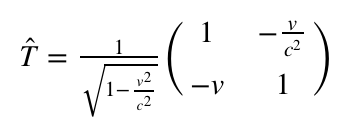# Deriving the Lorentz transformation

My last few posts have been all about visualizing the Lorentz transformation, the coordinate transformation in special relativity. But where does this transformation come from? In this post, I’ll derive it from basic principles. I saw this derivation first probably a year ago, and have since tried unsuccessfully to re-find the source.  It isn’t the algebraically simplest derivation I’ve seen, but it is the conceptually simplest. The principles we’ll use to derive the transformation should all seem extremely obvious to you.

So let’s dive straight in!

The Lorentz transformation in full generality is a 4D matrix that tells you how to transform spacetime coordinates in one inertial reference frame to spacetime coordinates in another inertial reference frame. It turns out that once you’ve found the Lorentz transformation for one spatial dimension, it’s quite simple to generalize it to three spatial dimensions, so for simplicity we’ll just stick to the 1D case. The Lorentz transformation also allows you to transform to a coordinate system that is both translated some distance and rotated some angle. Both of these are pretty straightforward, and work the way we intuitively think rotation and translation should work. So I’ll not consider them either. The interesting part of the Lorentz transformation is what happens when we translate to reference frames that are co-moving (moving with respect to one another). Strictly speaking, this is called a Lorentz boost. That’s what I’ll be deriving for you: the 1D Lorentz boost.

So, we start by imagine some reference frame, in which an event is labeled by its temporal and spatial coordinates: t and x. Then we look at a new reference frame moving at velocity v with respect to the starting reference frame. We describe the temporal and spatial coordinates of the same event in the new coordinate system: t’ and x’. In general, these new coordinates can be any function whatsoever of the starting coordinates and the velocity v.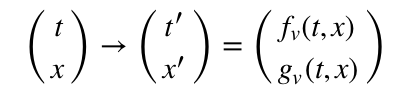To narrow down what these functions f and g might be, we need to postulate some general relationship between the primed and unprimed coordinate system.

So, our first postulate!

## 1. Straight lines stay straight.

Our first postulate is that all observers in inertial reference frames will agree about if an object is moving at a constant velocity. Since objects moving at constant velocities are straight lines on diagrams of position vs time, this is equivalent to saying that a straight path through spacetime in one reference frame is a straight path through spacetime in all reference frames.

More formally, if x is proportional to t, then x’ is proportional to t’ (though the constant of proportionality may differ).This postulate turns out to be immensely powerful. There is a special name for the types of transformations that keep straight lines straight: they are linear transformations. (Note, by the way, that the linearity is only in the coordinates t and x, since those are the things that retain straightness. There is no guarantee that the dependence on v will be linear, and in fact it will turn out not to be.)

These transformations are extremely simple, and can be represented by a matrix. Let’s write out the matrix in full generality: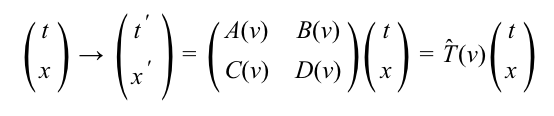We’ve gone from two functions (f and g) to four (A, B, C, and D). But in exchange, each of these four functions is now only a function of one variable: the velocity v. For ease of future reference, I’ve chosen to name the matrix T(v).

So, our first postulate gives us linearity. On to the second!

## 2. An object at rest in the starting reference frame is moving with velocity -v in the moving reference frame

This is more or less definitional. If somebody tells you that they had a function that transformed coordinates from one reference frame to a moving reference frame, then the most basic check you can do to see if they’re telling the truth is verify that objects at rest in the starting reference frame end up moving in the final reference frame. And again, it seems to follow from what it means for the reference frame to be moving right at 1 m/s that the initially stationary objects should end up moving left at 1 m/s.

Let’s consider an object sitting at rest at x = 0 in the starting frame of reference. Then we have: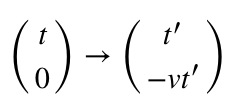We can plug this into our matrix to get a constraint on the functions A and C:Great! We’ve gone from four functions to three!## 3. Moving to the left at velocity v and to the right at the same velocity is the same as not moving at all

More specifically: Start with any reference frame. Now consider a new reference frame that is moving at velocity v with respect to the starting reference frame. Now, from this new reference frame, consider a third reference frame that is moving at velocity -v. This third reference frame should be identical to the one we started with. Got it?

Formally, this is simply saying the following: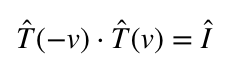(I is the identity matrix.)

To make this equation useful, we need to say more about T(-v). In particular, it would be best if we could express T(-v) in terms of our three functions A(v), B(v), and D(v). We do this with our next postulate:

## 4. Moving at velocity -v is the same as turning 180°, then moving at velocity v, then turning 180° again.

Again, this is quite self-explanatory. As a geometric fact, the reference frame you end up with by turning around, moving at velocity v, and then turning back has got to be the same as the reference frame you’d end up with by moving at velocity -v. All we need to formalize this postulate is the matrix corresponding to rotating 180°.There we go! Rotating by 180° is the same as taking every position in the starting reference frame and flipping its sign. Now we can write our postulate more precisely:Now we can finally use Postulate 3!Doing a little algebra, we get…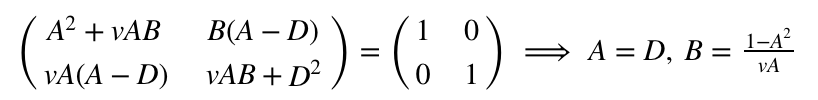(You might notice that we can only conclude that A = D if we reject the possibility that A = B = 0. We are allowed to do this because allowing A = B = 0 gives us a trivial result in which a moving reference frame experiences no time. Prove this for yourself!)

Now we have managed to express all four of our starting functions in terms of just one!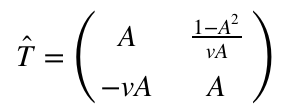So far our assumptions have been grounded by almost entirely a priori considerations about what we mean by velocity. It’s pretty amazing how far we got with so little! But to progress, we need to include one final a posteriori postulate, that which motivated Einstein to develop special relativity in the first place: the invariance of the speed of light.

## 5. Light’s velocity is c in all reference frames.

The motivation for this postulate comes from mountains of empirical evidence, as well as good theoretical arguments from the nature of light as an electromagnetic phenomenon. We can write it quite simply as:Plugging in our transformation, we get: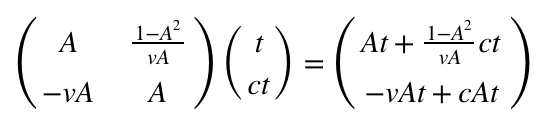Multiplying the time coordinate by c must give us the space coordinate:And we’re done with the derivation!

Summarizing our five postulates: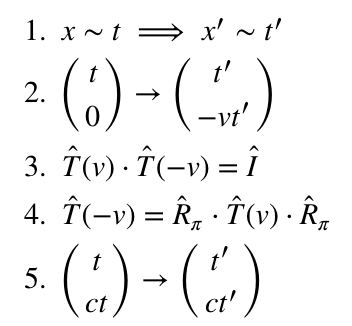And our final result: